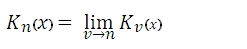# SQL Server BESSELK function

Updated: 27 August 2010

Use BESSELK function to calculate the Bessel function of the first kind Kv(z). For non-integer v, the BESSELKfunction is defined byIn the case of integer order n, the function is defined by taking the limit as non-integer v tends to nSyntax
SELECT [wctEngineering].[wct].[BESSELK] (
<@x, float,>
,<@n, float,>)
Arguments
@x
is the value at which to evaluate the function. @x is an expression of type float or of a type that can be implicitly converted to float
@n
is the order of the Bessel function. @n is an expression of type float or of a type that can be implicitly converted to float.
Return Types
float
Remarks
·         @n is truncated to zero decimal places.
·         If @n is less than zero, BESSELK returns an error
Examples

select
wct.BESSELK(2.5,1)

This produces the following result

----------------------
0.0738908156502669

(1 row(s) affected)

### SupportCopyright 2008-2023 Westclintech LLC         Privacy Policy        Terms of Service